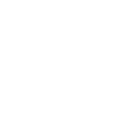×4th Chapter

### 12th Class Mathematics Chapter 4 Test

Here you can prepare 12th Class Mathematics Chapter 4 Introduction to Analytic Geometry Test. Click the button for 100% free full practice test.

## 12th class Mathematics chapter 4 online mcq test with answers for Fa part 2 Mathematics Chapter 4 (Introduction to Analytic Geometry)

This online test contains MCQs about following topics:.

- Introduction - Translation and Rotation of Axes - Equations of Straight Lines - Two and Three Straight Lines - Angles Between Two Lines - Homogeneous Equations-Second Degree in2 Variables

FA Part 2 Mathematics Ch 4 Test### 12th class Mathematics chapter 4 online mcq test with answers for Fa part 2 Mathematics Chapter 4 (Introduction to Analytic Geometry)

1
• A. Line parallel to x-axis
• B. Line parallel to y-axis
• C. Line passing through the origin
• D. Both (a) and (b)
2 The point of intersection of the perpendicular bisectors of a triangle is called:
• A. Centroid
• B. Ortho-center
• C. Circums-center
• D. In-center
3 The point of intersection of the medians of a triangle is called:
• A. Centroid
• B. Ortho-center
• C. Circums-center
• D. In-center
4 If the inclination of a line lies between ]90°, 180°[, then the slope of line is :
• A. Positive
• B. Negative
• C. Zero
• D. undefined
5 y = mx + c is the equation of straight line in:
• A. Slope-intercept form
• B. Two points from
• C. Point slope form
• D. Intercepts form
6 If in the case of translation of axes, O (-3, 2), (x, y) = (-6, 9) then (X, Y) =
• A. (-3, 9)
• B. (-3, 7)
• C. (-9, 11)
• D. (3, 7)
7 Point of intersection of lines x - 2y + 1 = 0 and 2x - y + 2 = 0 equals:
• A. (1, 0)
• B. (0, 1)
• C. (-1, 0)
• D. (0, -1)
8 The ratio in which x-axis divides the line segment joining the points:
• A. 1 : 1
• B. 1 : 3
• C. 1 : 5
• D. 1 : 2
9 x = 4 is a line:
• A. Parallel to x - axis
• B. Parallel to y - axis
• C. Perpendicular to y-axis
• D. None of these
10 The ratio in which the line segments joining (2, 3) and (4, 1) is divided by the line joining (1, 3) and (4, 3) is:
• A. 2 : 1
• B. 3 : 1
• C. 1 : 2
• D. 1 : 1

### Top Scorers of FSC Part 2 Mathematics Chapter 4 Test Online

D

#### Dileep Moolani

Lahore05 - Jul - 2023

12/20
08 Mins 51 Sec
D

#### Dileep Moolani

Lahore05 - Jul - 2023

10/20
16 Mins 16 Sec

Sort By:
X

to continue to ilmkidunya.comFill the form. Our admission consultants will call you with admission options.

X

to continue to ilmkidunya.com

X

to continue to ilmkidunya.com

X